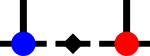SyTen## ◆ prodD() [4/6]

template<Rank r, typename Scalar >
 Scalar syten::CudaDenseTensorImpl::prodD ( CudaDenseTensor< r, Scalar > const & a, DenseTensor< r, Scalar > const & b, std::array< int, r > const & c_a, std::array< int, r > const & c_b, bool conjugate = false )
inline

Reordering scalar product of a CUDA dense tensor and a standard dense tensor.

If either tensor is larger than SYTEN_CUDA_THRESHOLD, the calculation is done on the GPU, otherwise, the calculation is done on the host.Here is the call graph for this function: